# 5 1 The Coordinate Plane Vocabulary coordinate plane

• Slides: 115 -1 The Coordinate Plane Vocabulary coordinate plane x-axis y-axis origin quadrant ordered pair5 -1 The Coordinate Plane 5 A coordinate plane is a 4 plane containing a Quadrant II 3 horizontal number line, the 2 1 x-axis, and a vertical 0 number line, the y-axis. – 5 – 4 – 3 – 2 – 1 The intersection of these – 2 axes is called the origin. Quadrant III– 3 y y-axis Quadrant I x-axis x 1 2 3 4 5 Origin Quadrant IV – 4 – 5 The axes divide the coordinate-plane into four regions called quadrants, which are numbered I, III, and IV.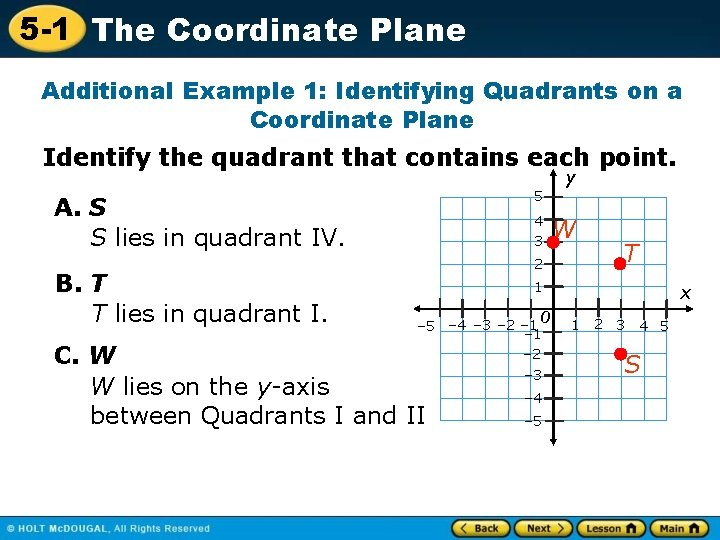5 -1 The Coordinate Plane Additional Example 1: Identifying Quadrants on a Coordinate Plane Identify the quadrant that contains each point. y 5 A. S S lies in quadrant IV. B. T T lies in quadrant I. 4 3 2 W T 1 0 – 5 – 4 – 3 – 2 – 1 – 2 C. W W lies on the y-axis between Quadrants I and II – 3 – 4 – 5 x 1 2 3 4 5 S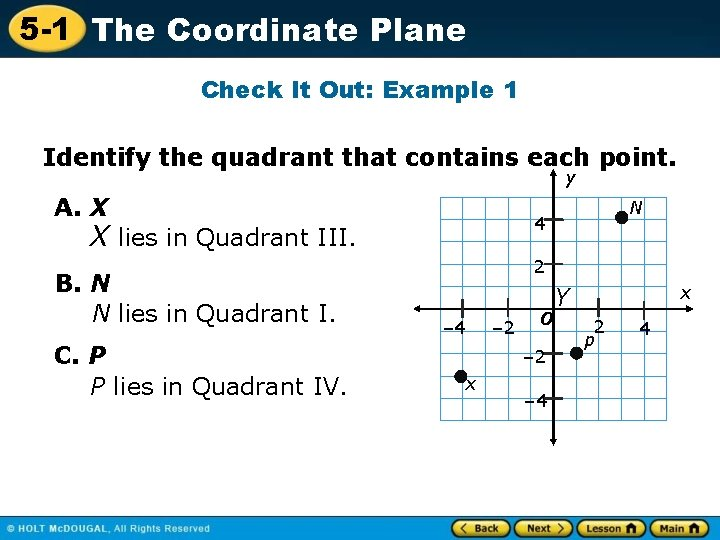5 -1 The Coordinate Plane Check It Out: Example 1 Identify the quadrant that contains each point. y A. X 4 X lies in Quadrant III. B. N N lies in Quadrant I. C. P P lies in Quadrant IV. N 2 – 4 – 2 0 – 2 x – 4 x Y 2 p 45 -1 The Coordinate Plane y Points on a coordinate plane are identified by ordered pairs. An ordered pair consists of two numbers in a certain order. The origin is the point (0, 0). 5 4 3 2 2 units up x 1 0 – 5 – 4 – 3 – 2 – 1 – 2 Ordered pair (3, 2) x-coordinate y-coordinate Units up Units right or left from 0 or down from 0 – 3 – 4 – 5 1 2 3 3 units right 4 5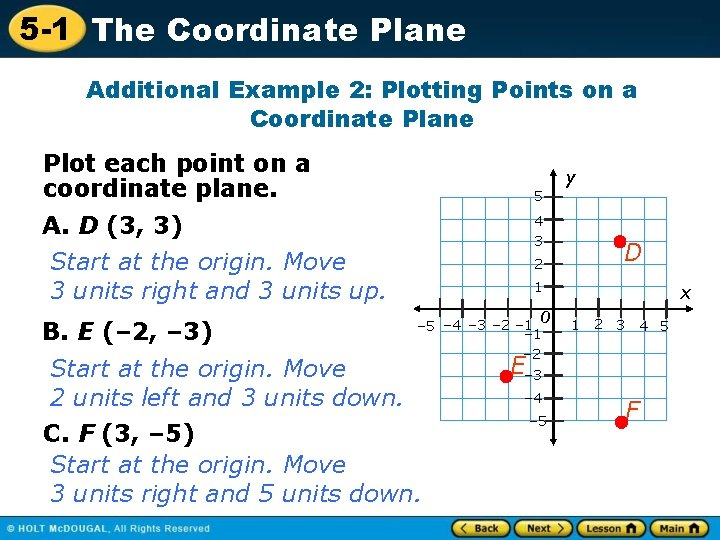5 -1 The Coordinate Plane Additional Example 2: Plotting Points on a Coordinate Plane Plot each point on a coordinate plane. y 5 A. D (3, 3) Start at the origin. Move 3 units right and 3 units up. B. E (– 2, – 3) 4 3 2 D 1 0 – 5 – 4 – 3 – 2 – 1 – 2 Start at the origin. Move 2 units left and 3 units down. C. F (3, – 5) Start at the origin. Move 3 units right and 5 units down. x 1 2 3 4 5 E– 3 – 4 – 5 F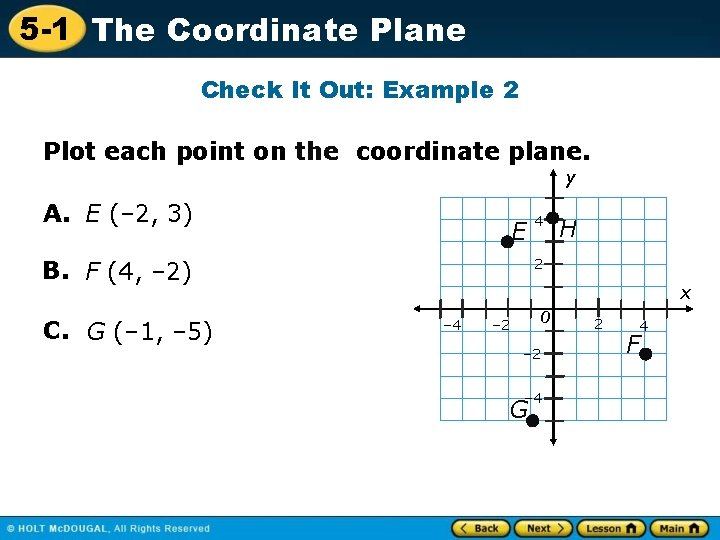5 -1 The Coordinate Plane Check It Out: Example 2 Plot each point on the coordinate plane. y A. E (– 2, 3) E H 2 B. F (4, – 2) C. G (– 1, – 5) 4 x – 4 0 – 2 – 4 G 2 4 F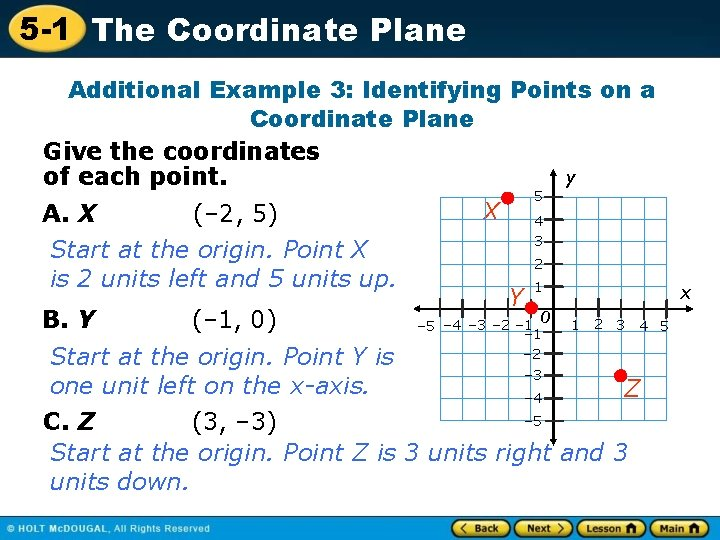5 -1 The Coordinate Plane Additional Example 3: Identifying Points on a Coordinate Plane Give the coordinates y of each point. 5 X 4 A. X (– 2, 5) Start at the origin. Point X is 2 units left and 5 units up. B. Y (– 1, 0) 3 2 Y 1 0 – 5 – 4 – 3 – 2 – 1 – 2 x 1 2 3 4 5 Start at the origin. Point Y is – 3 one unit left on the x-axis. Z – 4 – 5 C. Z (3, – 3) Start at the origin. Point Z is 3 units right and 3 units down.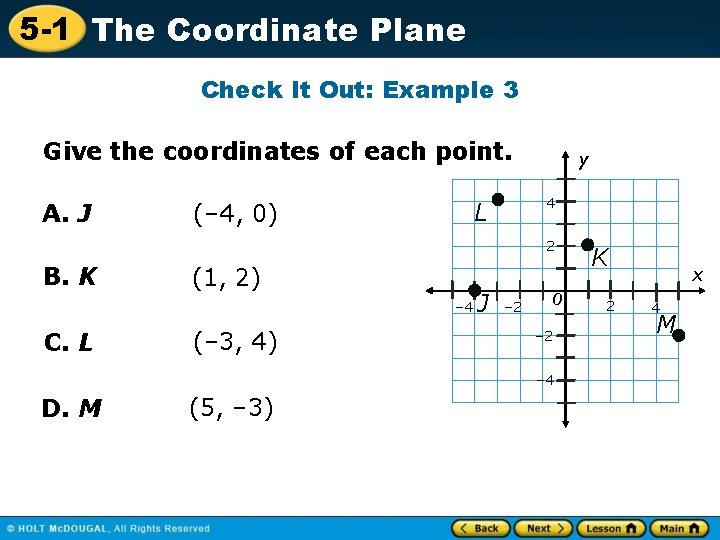5 -1 The Coordinate Plane Check It Out: Example 3 Give the coordinates of each point. A. J 4 L (– 4, 0) y 2 B. K (1, 2) – 4 C. L (– 3, 4) J – 2 0 – 2 – 4 D. M (5, – 3) K 2 x 4 M5 -1 The Coordinate Plane Lesson Quiz: Part I Give the coordinates of each point and identify the quadrant that contains each point y A 1. A (– 2, 4); II 2. B (3, – 2); IV 3. C (2, 3); I Plot each point on a coordinate plane. 4. D (2, – 3) 5. E (– 4, – 2) 5 4 3 C 2 1 0 – 5 – 4 – 3 – 2 – 1 – 2 E – 3 – 4 – 5 x 1 2 3 D 4 5 B5 -1 The Coordinate Plane Lesson Quiz: Part II 6. To plot (7, – 2) a student started at (0, 0) and moved 7 units left and 2 units down. What did the student do wrong? He should have moved 7 units right.